U.S. Department of Transportation
1200 New Jersey Avenue, SE
Washington, DC 20590
202-366-4000

Federal Highway Administration Research and Technology
Coordinating, Developing, and Delivering Highway Transportation InnovationsThis report is an archived publication and may contain dated technical, contact, and link information
 Federal Highway Administration > Publications > Research Publications > Geotechnicals > 04107 > Chapt2.Cfm > Behavior of Fiber-Reinforced Polymer Composite Piles Under Vertical Loads
 Publication Number: FHWA-HRT-04-107 Date: August 2006

# Behavior of Fiber-Reinforced Polymer Composite Piles Under Vertical Loads

## Chapter 2. Mechanical Short-Term Behavior of Frp Composite Materials Under Axial Compression Loads

The engineering use of FRP piles on a widespread basis requires developing and assessing reliable testing procedures and data analysis methods to establishing the material properties of the FRP composite piles. The results can be used to determine if these piles offer an alternative for deep foundation construction, especially in waterfront environments and aggressive soils.(1520) To address these engineering issues and assess the mechanical behavior of these materials under axial compression loads, FRP composite material specimens were tested in the laboratory. The composite material used in this study consisted of recycled plastic reinforced by fiberglass rebar (SEAPILE composite marine piles), recycled plastic reinforced by steel bars, and recycled plastic reinforced with randomly distributed fiberglass (Trimax).

### SEAPILE composite FRP material

In this chapter, the results of the laboratory compression tests performed on full-scale FRP composite material samples are discussed. The composite material used in this study consisted of recycled plastic reinforced by fiberglass rebar (SEAPILE composite marine piles) manufactured by Seaward International, Inc. The tests were performed on both the composite material specimens and each of the component materials, including the fiberglass bars and the recycled plastic.

The authors present an engineering analysis approach for establishing the equivalent mechanical properties of the composite material, including elastic modulus for the initial loading quasilinear phase, axial compression strength, inertia moment, and critical buckling load. The equivalent mechanical properties of the composite material are related to the mechanical properties of the component materials, assuming strain compatibility between the plastic and the fiber reinforcement during the axial compression loading. The results of this analysis illustrate that the main effect of the recycled plastic on the response of the composite material is to increase the buckling resistance. For the tested SEAPILE sample, the recycled plastic appears to prevent buckling of the bars but does not effectively prevent the peripheral disintegration of the fiberglass bars; therefore, it makes only a limited contribution to the axial compression strength of the composite material.

### Mechanical properties of the FRP composite and the component materials

SEAPILE composite marine piles consist of an extruded recycled plastic material reinforced by fiberglass bars (figure 9). The fiberglass rebar are manufactured in a pultrusion process, using fiberglass strands and polyester resin. The recycled plastic is composed mainly of high, medium, and low density polypropylene (HDPE, MDPE, and LDPE). It also contains a small percentage of polypropylene made from post-consumer waste (e.g., blow-molded bottles, plastic barrels, plastic bags, and TV cable jackets) and industrial waste (e.g., scrap pipes, telephone cables, film auto carpets, diaper trim, and auto insulation). The composite pile material consists of an inner core, foam that contains the fiberglass bars, and a skin. The core and the foam are made of recycled plastic, and the skin is made of a solid polyethylene compounded with pigments, ultraviolet (UV) absorbers, and antioxidants. The skin is relatively smooth, giving a friction coefficient of approximately 0.21 (wet) and 0.22 (dry), when tested in accordance with American Society for Testing and Materials (ASTM) F489.(21,22)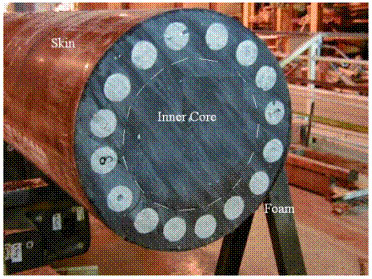Figure 9. Photo. SEAPILE composite marine piles.

To establish the mechanical properties of the fiberglass bars, two axial compression tests were performed on 4.4-cm- (1.75-inch-) diameter bars. The maximum load reached 591.6 kN (133 kips) and, at this point, the bars displayed a brittle failure. Figure 10 illustrates the stress-strain relationship of the fiberglass bars. Young's modulus of the fiberglass bars is 1.6x107 kPa (2,320 ksi), and the maximum stress at failure is 3.63x105 kPa (52.6 ksi). Figure 11 shows the bar both before and after parts of it disintegrated upon test completion. It illustrates that the failure mechanism involves peripheral disintegration by peeling fiberglass from the 9-cm- (3.54-inch-) long bar, which did not buckle during the loading. Considering the elastic properties of the bar, its buckling free length was calculated (according to Euler's equation) to be 22.5 cm (8.9 inches) for this failure load.Figure 10. Graph. Stress-strain relationship of 4.4-cm (1.75-inch) fiberglass bars.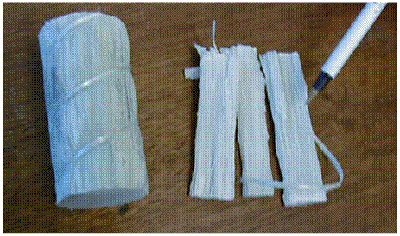Figure 11. Photo. Fiberglass bar before axial compression test and disintegrated fiberglass parts.

Axial compression tests were performed on the recycled plastic foam. The sample's dimensions were 15.2 cm (6 inches) in diameter and 30.4 cm (12 inches) long. Figure 12 shows the stress-strain relationships of the recycled plastic material obtained for strain rates of 0.1 cm (0.04 inch) per minute (min) (0.33 percent/min) and for 0.025 cm (0.01 inch) per min (0.083 percent/min). The results illustrate that the nonlinear response of this recycled plastic material to the axial loading is strain-rate dependent. Young's modulus of the recycled plastic obtained for the linear portion of the stress-strain curves are 3 x 105 kilopascals (kPa) (43.5 ksi) and 1.93 x 105 kPa (28 ksi), respectively, for the strain rates of 0.33 percent/min and 0.083 percent/min. Figure 13 shows the axial stress versus radial strain relationships obtained for the recycled plastic foam. The calculated Poisson's ratio is equal to 0.37, and the corresponding shear modulus value for the linear portion of the stress-strain curve is 109,489 kPa (15.9 ksi).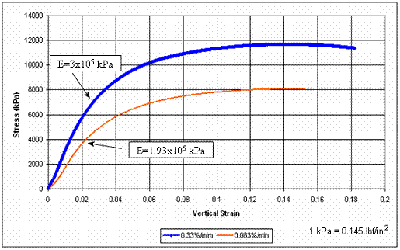Figure 12. Graph. Stress-vertical strain relationship of SEAPILE pile recycled plastic.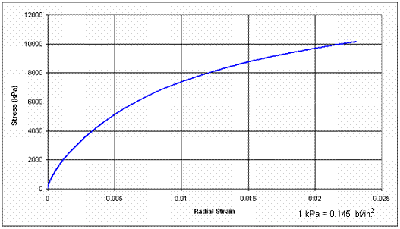Figure 13. Graph. Stress-radial strain relationship of SEAPILE pile recycled plastic; rate 0.33 percent/min.

To establish the composite material properties, a compression axial test was performed on a SEAPILE pile sample 40.6 cm (16 inches) in diameter and 81 cm (32 inches) long. The sample contained 16 fiberglass reinforcing rods each 7.6 cm (1.75 inches) in diameter. Because the top end of the sample was not leveled, Hydrostone® grout was placed between the pile upper surface and the loading steel plate. Three Geokon vibrating wire strain gauges were installed in the pile section on the fiberglass rebar, and four additional strain gauges were glued into holes on the outer skin. After the initial seating load was applied, the nominal loading rate was 0.25 mm (0.01 inch) per min.

Figure 14 shows the force-strain relationship obtained for the FRP composite material under the axial compression test. The maximum load reached was 9661 kN (2172 kips), at which point the sample experienced a brittle failure. The Young's modulus of the composite material was 3.38 x 106 kPa (490.23 ksi). Figure 15 shows the post-failure, full-scale sample section. It illustrates that the bars did not experience any buckling during loading, and only the upper section of the sample was damaged.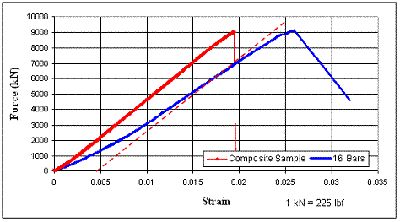Figure 14. Graph. Force-vertical strain relationship of SEAPILE pile sample.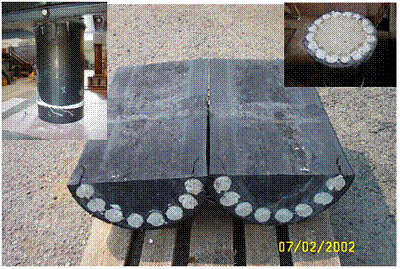Figure 15. Graph. SEAPILE pile sample after axial compression test.

### Engineering Analysis of the axial compression test on the composite pile sample

#### Elastic Modulus and Compressive Strength

The main purpose of the engineering analysis is to establish the equivalent mechanical properties of the composite material, including the elastic modulus for the initial loading phase, the axial compression strength, the inertia moment, and the critical buckling load. The equivalent material properties of the composite are related to the mechanical properties of the component materials, assuming strain compatibility between the plastic and the fiber reinforcement during the axial compression loading. The following assumptions were used in this analysis.

For the composite material section, the equilibrium equation under the applied load, F, can be written as in figure 16.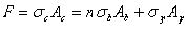Figure 16. Equation. Applied load F.

Where:

σc = Stress of the total section of the sample.

Ac = Cross-sectional area of the sample.

n = Number of bars in the composite section.

σb = Stress of the fiberglass bar.

Ab = Section area of the fiberglass bar.

σp = Stress of the plastic.

Ap = Section area of the plastic.

For axial compression, assuming elastic materials and strain compatibility between the plastic and the fiber reinforcement during the axial compression loading implies the relationships in figure 17.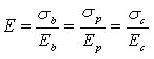Figure 17. Equation. Young's modulus E.

Where:

E = Young's modulus.

Eb = Young's modulus of the bar.

Ep = Young's modulus of the plastic.

Ec = Young's modulus of the total section of the sample.

Solving the equations in figures 16 and 17 yields the equation in figure 18.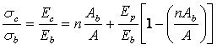Figure 18. Equation. σcb.

By making the following substitutions,

α = nAb/A, where α is defined as the area replacement factor,

β = Ep/Eb, where β is the relative axial stiffness coefficient,

Ac = A, where Ac is the section area of the composite sample,

the equation in figure 18 can be written in the form of the equation in figure 19.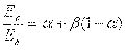Figure 19. Equation. Ec/Eb.

The equation in figure 19 yields the equivalent Young's modulus of the composite material as a function of the replacement factor α, the relative stiffness coefficient β, and the Young's modulus of the fiberglass bar Eb. For the SEAPILE composite material, equation 4 yields an equivalent Young's modulus value of Ec= 3.05 x 106 kPa (442.36 ksi); the difference between this calculated Ec value and the experimental result obtained for the composite sample Ec = 3.38 x 106 kPa (490.23 ksi) is less than 10 percent of the experimental value.

The equivalent shear modulus, Gc, of the composite material can be calculated from the theory of elasticity assuming that the FRP composite is an isotropic elastic material. This assumption does not take into account the anisotropy of the composite material induced by the preferential direction of the fiber reinforcement. Accordingly the equivalent shear modulus, Gc can be calculated by the equation in figure 20.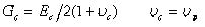Figure 20. Equation. Gc.

Where:

υc and υp are the Poisson's ratio values of the composite material and its recycled plastic component, respectively.

The equivalent compression strength, Rc, of the composite material is given by the equation in figure 21.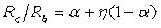Figure 21. Equation. Rc/Rb.

Where:

η = Rp/Rb, the compression strength ratio of the component materials.

Rp = Compression strength of the fiberglass bar.

Rb = Compression strength of the recycled plastic mobilized at the failure strain of the fiberglass bar (e = 2.6 percent).

For the SEAPILE composite material, the equation in figure 21 yields an equivalent compression strength Rc = 7.4 x 104 kPa (10.73 ksi); the difference between this calculated Rc value and the experimental result obtained for the composite sample, Rc = 6.8 x 104 kPa (9.86 ksi), is about 8.8 percent of the experimental value.

The effect of the recycled plastic on the behavior of the composite material can be analyzed considering the failure load factor (FLF), defined as the ratio of the failure load Fc of the composite material to the failure load nFb, defined as the failure load of the bar multiplied by the number of bars in the composite material. The FLF can be calculated by the equation in figure 22.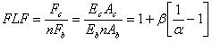Figure 22. Equation. FLF.

Figure 14 illustrates the experimental relationships between the applied axial load and the axial strain for the group of 16 bars and the composite material. The results show that, at failure by axial compression, the recycled plastic has only a limited effect on the load-bearing capacity of the composite material. The equation in figure 22 yields an FLF of 1.068, which is quite consistent with the experimental results (FLF = 1).

#### Moment of Inertia

Bending moment, assuming elastic materials and strain compatibility between the plastic and the fiber reinforcement during bending, implies the relationships in figure 23.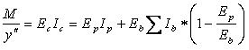Figure 23. Equation. Bending moment M.

Where:

M = Bending moment.

y″ = Second derivative of the displacement variation with depth.

SIb = Sum of the moments of inertia of all the fiberglass bars with respect to the axis of

symmetry of the composite material section.

Ip = Moment of inertia of the recycled plastic section with respect to the axis of symmetry of

the composite material section.

The moment of inertia (Ic) of the composite material can be derived by rearranging the equation in figure 23 to give the equation in figure 24.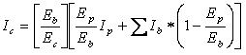Figure 24. Equation. Moment of inertia Ic(1).

By substituting β for Ep/Eb, the equation in figure 24 can be rewritten as the equation in figure 25.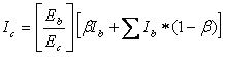Figure 25. Equation. Moment of inertia Ic(2).

The relative inertia moment coefficient λ is given by the equation in figure 26.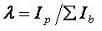Figure 26. Equation. Relative inertia moment coefficient λ.

Preceding equations can be combined and rearranged to give the equation in figure 27.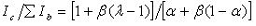Figure 27. Equation. Ic/ΣIb.

#### Critical Buckling Force

The critical buckling force, Pcr, can be calculated according to Euler's equation (figure 27).: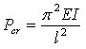Figure 28. Equation. Critical buckling force Pcr.

Where:

E = Young's modulus.

l = Length of the bar.

I = Moment of inertia.

For the fiberglass bar sample (E = 1.6 x 107 kPa (2,320.6 ksi); l = 9 cm (3.5 inches); I = 19.16 cm4 (0.46 inch4)), the equation in figure 28 yields the calculated critical buckling load of Pcr = 3,736 kN (839.8 kips). For a given fixed boundary condition at the top and at the bottom of the bar, k = 0.5, Pcr = 1,868 kN (419.9 kips). This buckling load exceeds the compression failure load of 591.6 kN (133 kips), causing the bar to collapse by peripheral disintegration.

The equivalent critical buckling load, Pcr, of an axially loaded bar confined in a low shear modulus material such as the recycled plastic is given by the equation in figure 29.(23)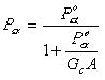Figure 29. Equation. Pcr of an axially loaded bar.

The term Pocr in figure 29 is the equivalent critical buckling load calculated for the composite material according to Euler's equation (figure 30).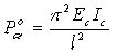Figure 30. Equation. Equivalent critical buckling load for composite material.

The terms Ec and Ic in figure 30 are the elastic Young's modulus and the moment of inertia of the composite material, respectively.

The effect of the recycled plastic on the buckling failure load of the composite material can be analyzed by considering the critical buckling load factor (BLF), defined as the ratio of the critical buckling load Pccr of the composite material to the critical buckling load of the bar, multiplied by the number of bars in the composite material ΣPbcr. The BLF can be calculated by the equation in figure 31.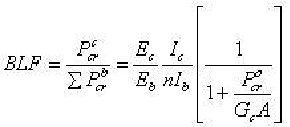Figure 31. Equation. Critical buckling load factor BLF.

Substituting λo = Ic /n Ib as a relative inertia moment index, the equation in figure 31 can be written as the equation in figure 32.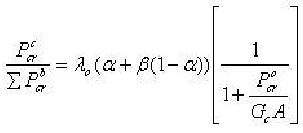Figure 32. Equation. Critical buckling load decomposed.

Figure 16 illustrates the variation of the critical buckling load with the length of the sample for the composite material and for the group of fiberglass bars. The critical buckling load of the composite material is calculated according to the equations in figures 29 and 30. The critical buckling load for the group of 16 bars is calculated from the sum of the buckling loads of the individual bars: ΣPbcr = n*Pbcr, where the critical buckling load of each bar, Pbcr, is calculated from the equation in figure 28. The results show that the recycled plastic has a significant effect on the critical buckling load.

The failure load of the sample is equal to the smaller load that can cause either buckling or peripheral disintegration of the pile matrix. It was observed that, for the failure load of 9,661 kN (2,172 kips), the composite material sample (40 cm (16 inches) in diameter and 80 cm (32 inches) in height) did not experience any buckling failure (figure 15). As illustrated in figure 33, under a critical buckling load which is equal to the compressive strength of the group of 16 bars, the calculated maximum free length is about 22 cm (8.7 inches), which is significantly smaller than the height of the composite sample. However, the maximum free length of the composite sample calculated from the equation in figure 29 for the composite material reaches about 150 cm (59 inches), illustrating that the recycled plastic appears to prevent buckling of the bars in the composite material. The critical BLF calculated from the equation in figure 31 for the composite sample reaches about 38, indicating the significant effect of the recycled plastic on the buckling resistance of the composite material.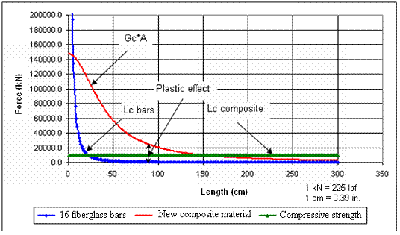Figure 33. Graph. Buckling force versus length for SEAPILE pile sample and 16 fiberglass bars.

### PPI Piles

PPI manufactures recycled plastic piles reinforced by steel bars (figure 34). The company obtains recycled plastic pellets from recycling firms that acquire their plastic from curbside collection and industrial waste. HDPE and LDPE are required to develop a cohesive and durable product. The formulation of the recycled plastic includes a mixture of approximately 30 percent HDPE and 70 percent LDPE. Carbon black material is added to the mix at the rate of 4 percent by weight of plastic to serve as a UV inhibitor. Celogen AZ130® is added at the rate of 0.5 percent by weight of plastic to encourage uniformity of porosity and minimize the size of voids. Calcium carbonate can be added to the mix to make the plastic material heavier when it is necessary to avoid a product that is lighter than water. The outer surface of the recycled plastic yields a material friction coefficient of approximately 0.20 (wet) and 0.22 (dry), when tested in accordance with ASTM F489.(24)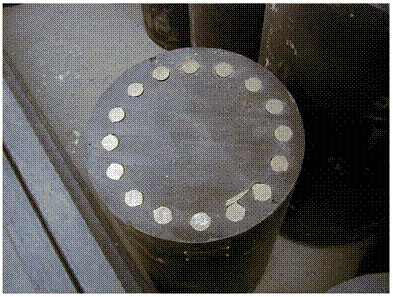Figure 34. Photo. PPI pile.

To establish the composite material properties, a compression axial test was performed on a PPI pile sample containing 16 steel reinforcing rods of 2.54-cm (1.0-inch) nominal diameter. The sample was 38.7 cm (15.25 inches) in diameter and 80 cm (31.5 inches) long. The results illustrate that this composite material has a behavior similar to that of steel (figure 35) and yields a compressive strength of 45,190 kPa (6.55 ksi). The Young's modulus of the composite material obtained for the linear portion of the stress-strain curve is 7.84 x 105 kPa (113.7 ksi).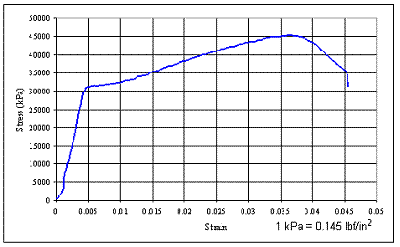Figure 35. Graph. PPI pile—stress-strain relationship.

### Trimax structural plastic material

Trimax structural plastic lumber material consists of recycled plastic, fiberglass, and selected additives (figure 36). The plastic raw material used in Trimax is derived from post-con sumer bottle waste such as milk and detergent bottles. This material is compounded into a consistent, reinforced plastic timber product using reactive compatibilizers, which create a stable plastic/fiber matrix.(25)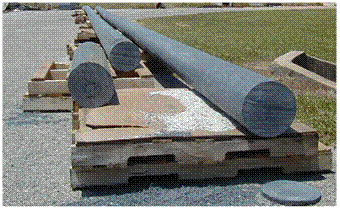Figure 36. Photo. Trimax pile.

#### Axial Compression Tests on the Trimax Pile Recycled Plastic

Axial compression tests were performed on the Trimax pile recycled plastic. The sample's dimensions were 25.4 cm (10 inches) in diameter and 50.8 cm (20 inches) long. Figure 37 shows the vertical stress-strain relationships obtained for several strain rates. The results illustrate that the nonlinear response of this recycled plastic material to the axial loading is strain-rate dependent. The Young's modulus of this material, obtained for the linear portion of the stress-strain curve at the strain rate of 1.7 mm (0.07 inch) per min (0.33 percent/min), is 370,000 kPa (53.7 ksi). Figure 38 shows the axial compression stress versus radial strain relationships obtained for strain rates of 0.33 percent/min. Poisson's ratio calculated for this strain rate at 1000 kPa (0.145 ksi) is equal to 0.35, and the corresponding shear modulus value for the linear portion of the stress-strain curve is 1.37 x 105 kPa (19.9 ksi).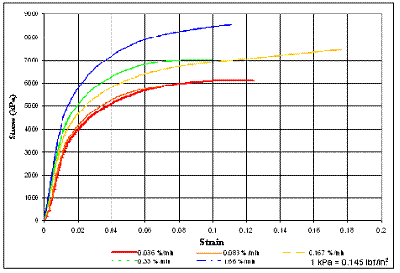Figure 37. Graph. Trimax pile—vertical stress-strain curves at different rates.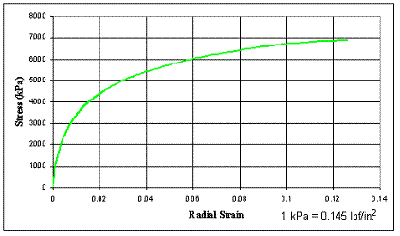Figure 38. Graph. Trimax pile—vertical stress-lateral strain curve; strain rate 0.33 percent per min.

Table 1 summarizes the comparison between the predicted and experimental values of the SEAPILE composite material properties.

Table 1. Material properties—test results and model calculations.
Material Property Recycled Plastic 16 Fiberglass Bars Composite Material Test Results Model Prediction
Elastic modulus, kPa (ksi) 300,000 (43.5) 1.6 x 107 (2,320) 3.38 x 106 (490.23) 3.05 x 106 (442.36)
Compressive strength, kPa (ksi) 1.169 x 104 (1.69) 3.63 x 105 (51.9) 6.8 x 104 (9.86) 7.4 x 104 (10.73)
Moment of inertia, m4 (inch4) 1.6 x 10-3 (3,844) 118.98 x 10-6 (285.8) - 0.77 x 10-3 (1850)
Critical buckling load, kN (kips) (80 cm) sample - 757 (170) 9661 (2172) (no buckling observed) 29,066 (6,534)